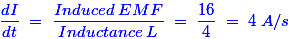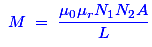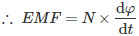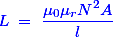# MCQs on Electromagnetic Fields

##### Page 7 of 20. Go to page 1 2 3 4 5 6 7 8 9 10 11 12 13 14 15 16 17 18 19 20
01․ Which of following circuit element stores energy in electro-magnetic field?
Inductor.
Condenser.
Variable Resistor.
Capacitor.

Inductor stores energy in the electro-magnetic field. Capacitor and condenser stores energy in the electrostatic field and resistance doesn’t store energy in any field.

02․ An emf of 16 volts is induced in a coil of inductance 4 H. The rate of change of current must be
64 A / s.
32 A / s.
16 A / s.
4 A / s.

The expression of the rate of change of current is given as03․ Which of following can induce the maximum induced voltage ?
1 amp dc.
1 amp 1 Hz.
1 amp 100 Hz.
20 amp dc.

If we apply a high frequency supply of the same peak voltage to the coil, the current still is being delayed by 90° but the time it requires to reach its maximum value has been reduced due to the increase in frequency. Because the frequency is inversely proportional to time (T). Hence, the rate of change of the flux within the coil has also increased due to the increase in frequency. Hence, the induced EMF is maximum in case of 1 amp 100 Hz supply source in the coil.

04․ The emf induced in a conductor rotating in bipolar field is
DC.
AC.
DC and AC both.
none of these.

It is nothing but the EMF in a conductor in a rotating magnetic field is sinusoidal and alternating. It may be observed from the process of EMF generation in a conductor. In the initial position, the conductor is parallel with magnetic flux lines. So, there is no EMF. When it reaches perpendicular to flux lines, EMF generation is maximum. After that again it reaches the parallel path to flux lines. So, EMF is zero now. This same action happens again, but it is in reverse direction. So, again there is an EMF but is in negative direction. Hence, we get the sinusoidal waveform that has a bipolar field and that is nothing but the AC.

05․ In case of all flux from the current in coil 1 links with coil 2, the coefficient of coupling will be
2.0.
1.0.
0.5.
Zero.

If all flux from the current in coil 1 links with coil 2, then that is referred as an ideal coupling. For an ideal coupling, the coefficient coupling is 1. Any other coupling the coupling co-efficient is always less than 1.

06․ Mutual inductance between two magnetically coupled coils depends on
permeability of core.
the number of their turns.
cross sectional area of their common core.
all of above.

The expression of the mutual inductance between two magnetically coupled coils is as follows;Where, μr = Relative permeability of core N = The number of the turns of the coil A = Cross sectional area of their common core Hence, the mutual inductance depends on all the above factors.

07․ The magnitude of the induced emf in a conductor depends upon on the
flux density of the magnetic field.
amount of flux cut.
rate of change of flux linkage.

According to the Faraday’s law of induction, the magnitude of the induced EMF is nothing but the product of the number of turns of the winding and the rate of change of flux linkage in it. Hence, the induced EMF in a conductor depends on the rate of change of flux linkage.08․ The property of the coil by which a counter emf is induced in it when the current through the coil changes is known as
self inductance.
mutual inductance.
series aiding inductance.
capacitance.

Self induction is that phenomenon in which a change in current or flux in that coil produces an induced emf in the coil itself. In mutual inductance the emf induced due to the flux linking of the other coil.

09․ A coil is wound on iron core which carries current I. The self-induced voltage in the coil is not affected by
variation in coil current.
Variation in voltage in the coil.
change the number of turns of coil.
the resistance of magnetic path.

Induced emf in a coil is always equal and opposite to the applied voltage across it. It does not depend upon the number of turns of the coil. If we increase the number of turns of the coil from 2 to 200 induced emf across the coil would still be same and opposite of the applied voltage.

10․ Both the number of turns and the core length of an inductive coil are doubled. Its self-inductance will be
unaffected.
doubled.
halved.